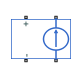# Voltage-Controlled Current Source

Linear voltage-controlled current source

## Library

Electrical Sources

•## Description

The Voltage-Controlled Current Source block models a linear voltage-controlled current source, described with the following equation:

`$I=K·\left(V\left(+\right)-V\left(-\right)\right)$`

where

 `I` Current `K` Transconductance `V(+)`,`V(-)` Voltages presented at the + and – control ports

To use the block, connect the + and – ports on the left side of the block (the control ports) to the control voltage source. The two ports on the right side of the block (the output ports) generate the output current. The arrow indicates the positive direction of the current flow.

### Variables

To set the priority and initial target values for the block variables prior to simulation, use the Initial Targets section in the block dialog box or Property Inspector. For more information, see Set Priority and Initial Target for Block Variables.

Nominal values provide a way to specify the expected magnitude of a variable in a model. Using system scaling based on nominal values increases the simulation robustness. Nominal values can come from different sources, one of which is the Nominal Values section in the block dialog box or Property Inspector. For more information, see Modify Nominal Values for a Block Variable.

## Parameters

Transconductance K

Transconductance, or the change in output current divided by the change in input voltage that causes it. The default value is `1` 1/Ω.

## Ports

The block has four electrical conserving ports. Connections + and – on the left side of the block are the control ports. The other two ports are the electrical terminals that provide the output current. The arrow indicates the positive direction of the current flow.

## Version History

Introduced in R2007a